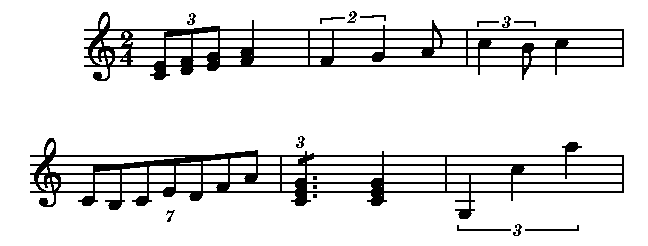Tuplets

Tuplets are specified by giving a list of chords within curly braces. The ending brace is optionally followed by a side ("above" or "below"). This is followed by a number or pair of numbers, and a semicolon.

The "side" tells Mup whether to print the tuplet number and bracket above or below the chords. It only applies on voice 1 or 2 when the other voice (1 or 2) is nonexistent or a space. If there are at least two voices, the number and bracket will always be put above on voice 1 and below on voice 2. You can always force the side on voice 3. If you don't specify a side for a tuplet, Mup will choose the side that seems best.

The first number is the number that should be printed with the tuplet. If it is followed by an "n," the number (and bracket) will not actually be printed. If it is followed by a "y," the number and bracket will always be printed, unless there is only a single chord in the tuplet, in which case only the number will be printed. If it is followed by "num," the number will always be printed, but the bracket will never be printed. If none of those modifiers are specified, the number will always be printed, but the bracket will be omitted in cases where all the notes in the tuplet are beamed together and the beam does not include any notes not in the tuplet.

The second number, if any, is separated from the first by a comma, and tells the time unit that the tuplet is to take up. This number can be dotted if necessary. It can also be a time expression, like 2+8, although that is very rarely likely to be useful. If no second number is given, the default is to fit in the next shorter un-dotted note. For example, three eighth notes would be fitted into the time of a quarter note, or five eighth notes would be fitted into the time of a half note.

Occasionally in music, as a shorthand, a single note or chord is printed with a tuplet number to indicate the note or chord is to be repeated several times as a tuplet. Mup will allow this; simply use a tuplet with only one chord, and add one or more slashes.

The final item that can optionally be specified is the word "slope," followed by an angle in degrees, for the the angle of the tuplet bracket, if any. If that is omitted, the angle is calculated based on the tupletslope parameter.

Nested tuplets are not allowed.

Here are some examples of tuplets:

// This has a triplet, where 3 eighth
// notes take as much time as a
// normal quarter note.
1: { 8ce; df; eg;}3; 4fa;
bar

// This has a dublet, in which 2 quarter notes
// take the time of a normal dotted quarter
1: { 4f; g; } 2, 4.;8a;
bar

// A quarter note and eighth note that
// make up a triplet the length of a
// normal quarter note,
// with the 3 printed above the notes
1: { 4c+; 8b; } above 3; 4c+;
bar

// A septuplet in the time of a half note
// with the "7" printed below the notes
1: { 8c; b-; c; e; d; f; a; } below 7;
bar

// A single chord tuplet
1:  { [slash 1] 4.ceg;}3; 4;
bar

// Forcing a tuplet bracket to be flat
1: {g-;c+;a+;}3 slope 0;
bar